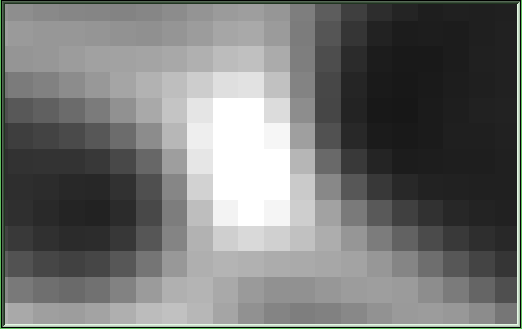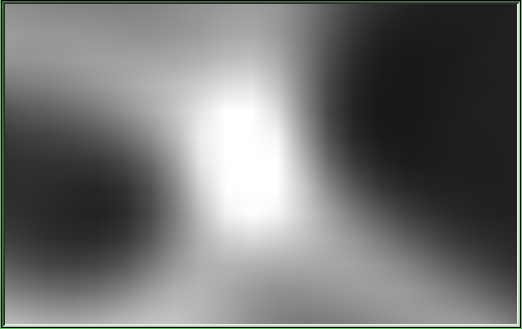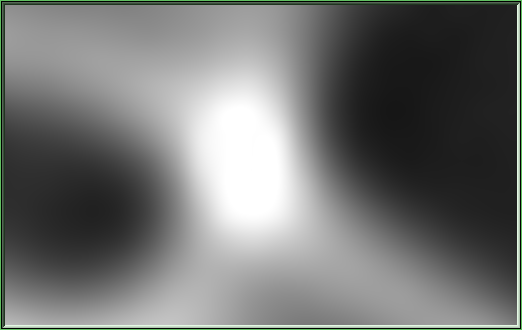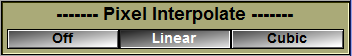Tool: 2D Pixel Interpolation

With this tool, you can interpolation to smooth out the GLI pixels when the image is magnified.Pixel Interpolation: Off Pixel Interpolation: Linear Pixel Interpolation: Cubic

Note:

 To interpolation is only displayed at high magnification.  If the image magnification is inferior to \$INTERPOLATION_MIN (default=2) the interpolation is not displayed.

Note:

 To interpolation will interfere with the "Mode Thresholding" visualization.

Note:

 To interpolation will severely decrease the image refresh rate.

From the Graphic InterfaceOff / Linear / Cubic These  buttons change the interpolation mode.  •Off turn it off •Linear use a bilinear interpolation based on the pixels immediate neighbours. •Cubic use a Catmull-Rom cubic spline to interpolate from a matrix of 4x4 pixels.

From the Display Area

There is no Display Area interaction specific to this tool.

From the Keyboard

The following commands can also be mapped to keyboard keys as a shortcut:

 Key map Action "i" Toggle the interpolation mode between Off and Linear.

From the Command Line

System Variables defined in this library:

 \$INTERPOLATION_MODE (U8) Current interpolation mode: 0=off, 1=linear, 2=cubic (Default = 0) \$INTERPOLATION_MIN (U8) Minimum magnification for interpolation (Default = 2)

Commands recognized in this library:

Interpolation: Mode (Off | Linear | Cubic | Toggle)

Set the current interpolation mode:

Off = Disable interpolation (\$INTERPOLATION_MODE=0).

Linear = Enable bi-linear interpolation (\$INTERPOLATION_MODE=1).

Cubic = Enable bi-cubic interpolation (\$INTERPOLATION_MODE=2).

Toggle = Toggle between modes off and linear.